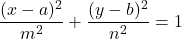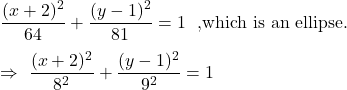Question

what is the length of the major axis of the conic section (x+2)^2/64+(y-1)^2/81=1

1.RuslanHeatt

Answer: The length of the major axis = 18 units.

Step-by-step explanation:

For equation ellipse:If n>m , then length of major axis = 2n

Otherwise 2m

Given equation:9>8

So, the length of the major axis = 2(9) = 18 units.